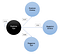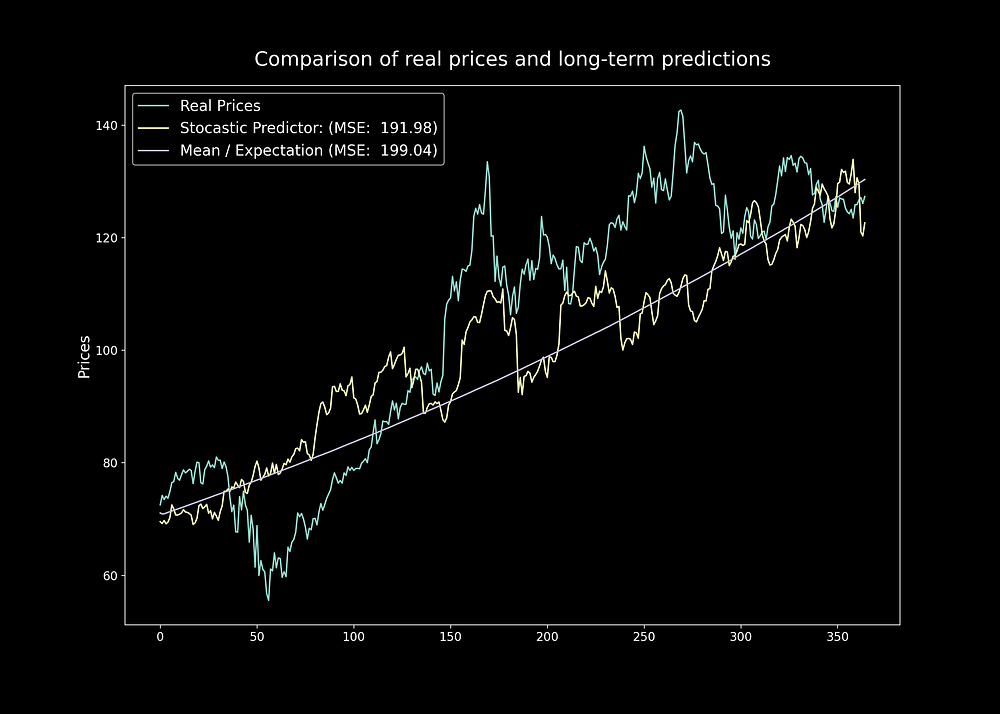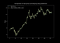# Retiring Regression with Simulated Evolution

Time-series problems or problems with numerical targets are often related to Regression, that’s how many have been taught. A recent experience had me considered “retiring regression”, this is certainly an overstatement, but it is worth the thinking because, as one of the oldest and most commonly used techniques, Regression has some fundamental flaws for its use. Here are TWO commonly met problems in Regression:

1. Regressors are terrible at extrapolation.

The name “regression” tells a story that relational data points tend to lean towards their expectation, and their velocity of doing so depends very much on how extreme the previous data point was against the expectation. Interpolation, which regression excels at, is about making inference inside the space covered by the sample observations, whereas extrapolation is inferencing outside the space. When the expectation is fixed, regression is great at mapping the relationships between variables, assuming that there is enough data to cover major possibilities. But if the expectation is non-stationary, there will never be enough data at hand as the expectation is ever-changing, which leads to overfitting at every step of the way. Not that there is no way to make Regressors better at extrapolation, it’s just that by design regression is not suitable for extrapolation.

2. Regressors have limited capacity.

The second problem, which is related to the first one, is about the resources that Regressors can maneuver. Powerful Deep Regressors like RNN are sometimes not worth the trouble; they are not only hard to fine-tune, but also suffer from “vanishing gradients” that limits not only their ability to look forward into the future, but also looking backwards into the past. The longer the look back period, (1) the longer it takes to train, (2) the deeper the vanishing gradients, and (3) the more data is needed. Though, with attention models, the first 2 issues can be adequately addressed, but the lack of data remains a headache because time series is not expandable (i.e. we cannot ask time to repeat itself, so that we can collect more data).

# Alternative: Simulation as prediction

The two problems of regression had us look for other alternatives. There are usually two ways to understand a dynamical system: “Formulation” and “Simulation”, and the rise of Generative models (e.g., GAN) prompts a third method: “Generation”. Formulation (e.g., regression) requires a rigorous mathematical proof for a theory, such as an equation, to be used as first principle to mimic the system behavior. When the first principle is not available, Simulation (e.g., Monte Carlo) allows us to collect samples of the system behavior and come up with some rules to mimic the system behavior. Simulation doesn’t aim at understanding the system itself, it merely focuses on its behavior on a surface level. Many complex systems, such as Quantum Physics, are not explainable at the moment, but knowing their behaviors is enough for us to build wonderful applications. At the core, simulation is prediction, because there is no use in simulating the wrong system behavior. “Generation” is a hybrid between formulation and simulation, but it is a topic for another day.

Below we will explore a simulation-based regression alternative; the model we are about to build is nicknamed “SE1”, short for Simulated Evolution, and 1 indicates its base form (the simplest version of this idea).

We will use stock price prediction as a problem to demonstrate the idea of Simulation as Prediction. The objective here is to minimize the Mean Squared Error on test data. Firstly, let’s grab the data, we will use Apple Inc. as an example, and get the data from Yahoo Finance.

The above code block returns a data frame with 3 features: dates, close prices, and the % changes of the close prices. Next, we use the most recent 2 years of data (a total of 365 * 2 time steps) to make our training data and test data.

If this is treated as a regression task, we would train our Regressor on training data (0- 365 time steps) and test it on test data (365–730 time steps). In our case, we want to make a simulator out of this time series, and the way to do so is by decomposing this time series with its local dynamics in order to make different stochastic environments.

There are many methods of decomposition, such as unsupervised learning, but we will do a more simple but effective decomposition on ‘%Change’ column. If you wonder, why use ‘%Change’ and not ‘Close’ prices: Predicting ‘%Change’ allows us to simulate data independent of the initial starting point, and we can always recover the predicted next price given the previous price and the predicted change:

As far as decomposition is concerned, we will group the changes into FOUR groups / environments. At any given time, a % change can be either positive or negative, and also going up or down.

Based on these four groups / environments, we will define a dictionary to hold samples for each one as shown below:

The list in each environment should contain either float values or lists of float values (consecutive points may be added to the same group at a time). Here we will also define the transitional counts from one environment to another environment, which we will later use to compute transitional probabilities between environments:

Computing transitional probabilities is easy: We just have to count through our training data points as we classify them into different environments:

The resulting transitional probabilities come from counts: probability(x) = x /(sum(all_x). Notice that some samples will never follow each other; for example, if ‘PositiveUp’ already took place, ‘NegativeUps’ will not follow because the next data has to be in ‘NegativeDowns’ first.

So far, we have obtained two things: (1) The samples for each environment and (2) the transitional probabilities between those environments. Now we can finally construct a Markov Chain to be used as a simulator:Starting at ‘PositiveUps’, there are three possible directions to move onto.

The diagram below shows 100 of the 50,000 simulations conditional on the last value of the training data, each simulation has 365 time steps as 365 is the length of the test data.

Both empirical and experimental evidence shows that the more simulations the better, because there is a higher chance that a better simulation can be found. The simulation process can be done on thread/core-based parallelism, much similar to evolutionary algorithms, which gives SE1 a lot of room for optimization.

# A. Far-sighted PredictionMean is the average of all simulated prices and Stochastic Predictor is the simulation closest to the mean.

Long-shot prediction is possible with SE1, because out of all the simulations, there is at least one that is very close to truth; the problem is just to find it. Here we will take a more empirical approach: After taking the mean(expectation) of all simulations, we will select the simulation closest to the mean based on MSE, and then put the two against the test data. The expectation is very much like a regression line, but this line is shown on held-out data, the simulations are thus making the kind of extrapolation that seems to be as good as a regression making interpolation. This long-term prediction conditional only on one true value is far from perfect, but it has already performed better than a regular Regressor.

# B. Short-sighted PredictionThe above results combines 100 simulations (N = 100) for each time step and uses a validation period of 7 (N = 7)

To do predictions step-by-step, we can select, at each point, the N simulations that have the smallest MSE on the past M days, and then combine the predictions of those simulations. The two parameters N and M are important: If the training is not representative, we should use smaller N to focus more on the best performing simulation, and vice versa. The look back period M can be considered as an ongoing validation period; it needs only to be a small number, such as 1–7. With more fine-tuning, the prediction is not bad at all!

# Concluding Summary

1. SE1 is great at extrapolation and enjoys long predictive span. A particular reason why SE1 is better suitable for extrapolation is because we are dealing with a Search problem, rather than a prediction problem, where among the 50,000 possibilities we have simulated, there is at least one that is extremely close to the ground truth.
2. SE1 is easy since there is no gradients involved; the model is lightweight (as it’s essentially non-parametric) and versatile (as we can obtain however many predictions we desire). Because it’s a simulator and predictor, it also addresses the problem of data shortage in time series.
1. Reinforcement Learning & Evolutionary Strategies: The reason why I constantly use the term “Environments” rather than “Groups” or “Classes” to describe the decomposed local dynamics is because we can consider the evolution of a time-series as, you might have guessed it, evolution. An agent can be rewarded with +1 if it successfully transitions to another environment by meeting a pre-set target; the length of a sample in a particular environment defines how long the environment will stay its course before it evolves to another environment. A simulator like SE1 provides a convenient way for a Reinforcement Learning agent to adapt to the changing environments.
2. Improving Regressor: The simple way to improve SE1’s predictability is to couple it up with a Regressor. Since SE1 is also a simulator, it can also address Regressor’s data shortage problem and helps it avoid overfitting.

Focusing on RL/Generative Models and beyond. This is a medium for blogging in AI & Compute and networking.

Focusing on RL/Generative Models and beyond. This is a medium for blogging in AI & Compute and networking.